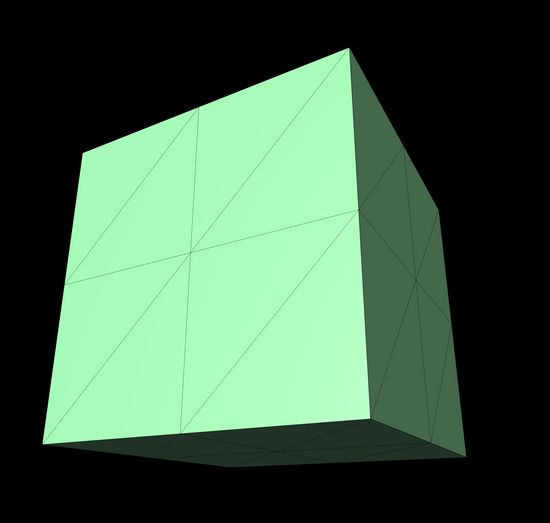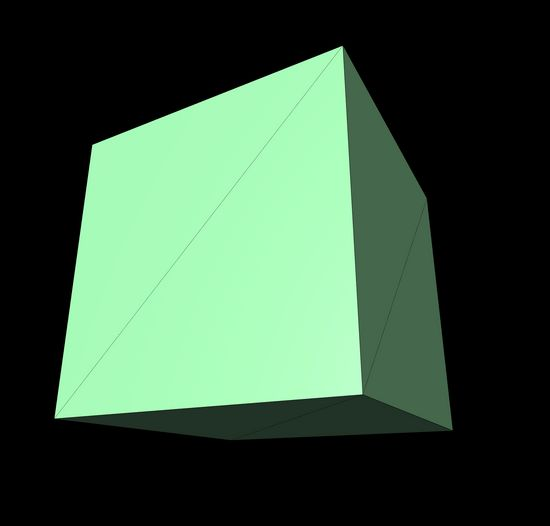# Understanding & modifying vertex positions in ThreeJS

Last updated: Jul 2, 2023

I've been on-and-off, slowwwwly, learning more about ThreeJS and 3D graphics creations. This note originated with me wanting to grab all the corners of a shape like a cube, and add randomness to them to create a fun/funky shape. I ended up digging into how geometries are constructed in ThreeJS and how to create and manipulate the vertices of a particular shape.

Every geometry in ThreeJS is made up of a bunch of segments. Segments are basically lines that divide each face of the shape into triangles.

Segments are set when creating a geometry, like so...

``const r = 5const widthSegments = 1const heightSegments = 1const depthSegments = 1const geometry = new THREE.BoxGeometry(r, r, r, widthSegments, heightSegments, depthSegments)``

Here is a box geometry created with 2 segments in each direction:But the default is one per direction, which looks like this:So let's say we start out with the simplest shape, with one segment in each direction. Each of these geometries has a `positions` attribute listing all of its vertex positions, which we can get like this:

``const positions = geometry.getAttribute('position') as THREE.BufferAttributeconst count = positions.count``

If you `console.log(positions)` here you'll see an object with a few attributes. One is an array with a length of 72. The more important part is actually `positions.count` which in this case is 24. This means the shape has 24 vertices.

The 24 comes from 1) the shape has 6 faces, and 2) each face has 4 individual points (vertices). I was super confused for a long time because I thought the points were based on how many triangles were in each face, and trying to find some kind of way to multiple 3 points per triangle and 6 faces to get to the right number. But nope.

`positions.array` has 72 values because each vertex has an x, y, and z value - meaning 24 * 3 = 72 individual values in our array.

If we go back to our shape with 2 segments each way, `positions.count` is 54. 9 points/vertices on each face, multipled by 6 faces.

We can get a particular point from this positions array and modify it like this:

``let n = 0 // position indexlet point = new THREE.Vector3()point = point.fromBufferAttribute(positions, n)// modify the positionlet x = point.x * Math.random()let y = point.y * Math.random()let z = point.z * Math.random()positions.setXYZ(n, x, y, z)``

But if we just loop through the points and randomize the values on all of them, it looks like this, which is not what we want:

It's... not really a shape anymore. The problem is that many of the vertices in `positions` are duplicated. The corners of each face of the shape are also corners of another face. For our shape to stay an intact shape, those vertices need to stay the same as each other.

Instead, we can create a simple map to store each position as we iterate through them. On each vertex, we check - have we seen this position before? If we haven't, great, we create some new randomized values and set the position to those values. But if we have, we remind ourselves of the randomized values we already used, and just update the current vertex to match.

``let vary = 1.5 // or whateverconst positions = geometry.getAttribute('position')const count = positions.countlet verticesMap = {}let point = new THREE.Vector3()for (let i = 0; i < count; i++) {	point = point.fromBufferAttribute(positions, i)	let key = [point.x, point.y, point.z].join(',')	if (!verticesMap[key]) {		verticesMap[key] = {			x: point.x + Math.random() * vary,			y: point.y + Math.random() * vary,			z: point.z + Math.random() * vary,		}	}	let { x, y, z } = verticesMap[key]	positions.setXYZ(i, x, y, z)}geometry.computeVertexNormals() // update colors & shading``

It works! Have a funky shape: